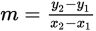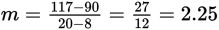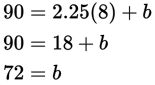# SAT Math Multiple Choice Question 594: Answer and Explanation

### Test Information

Question: 594

9. Human beings have a resting heart rate and an active heart rate. The resting heart rate is the rate at which the heart beats when a person is at rest, engaging in no activity. The active heart rate rises as activity rises. For a fairly active woman in her 20s, eight minutes of moderate exercise results in a heart rate of about 90 beats per minute. After 20 minutes, the same woman's heart rate will be about 117 beats per minute. If the human heart rate increases at a constant rate as the time spent exercising increases, which of the following linear models represents this same woman's heart rate, r, after t minutes of moderate exercise?

• A. r = 0.15t – 5.3
• B. r = 0.44t – 32
• C. r = 2.25t + 72
• D. r = 6.75t + 36

Explanation:

C

Difficulty: Medium

Category: Heart of Algebra / Linear Equations

Strategic Advice: You'll need to interpret the information given in the question to write two ordered pairs. Then you can use the ordered pairs to find the slope and the y-intercept of the linear model.

Getting to the Answer: In an ordered pair, the independent variable is always written first. Here, the heart rate depends on the amount of exercise, so the ordered pairs should be written in the form (time, heart rate). They are (8, 90) and (20, 117). Use these points in the slope formula,, to find that. Then, substitute the slope (2.25) and either of the points into slope-intercept form and simply to find the y-intercept:Finally, write the equation using the slope and the y-intercept that you found to get r = 2.25t + 72. Note that the only choice with a slope of 2.25 is (C), so you could have eliminated the other three choices before finding the y-intercept and saved yourself a bit of time.